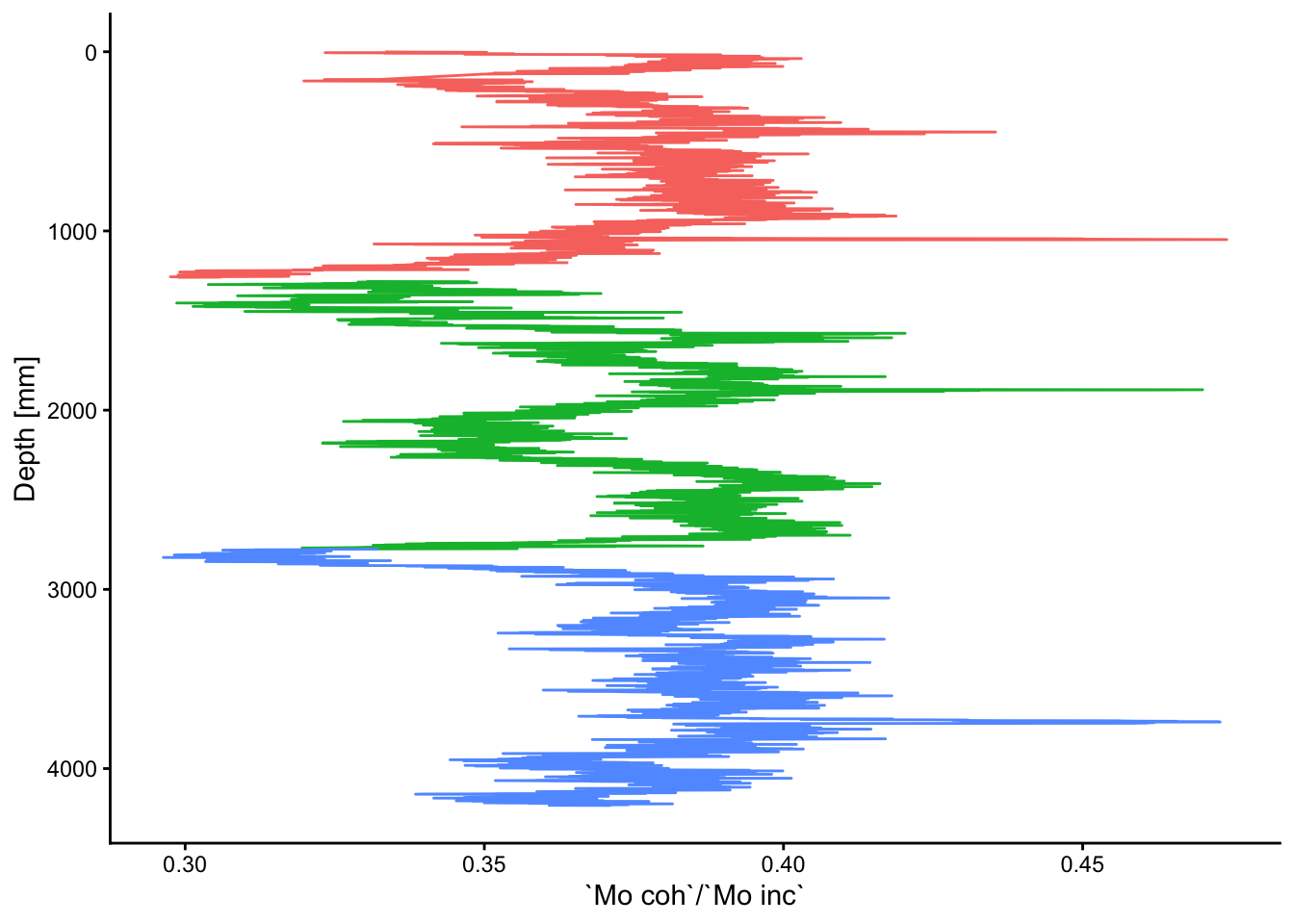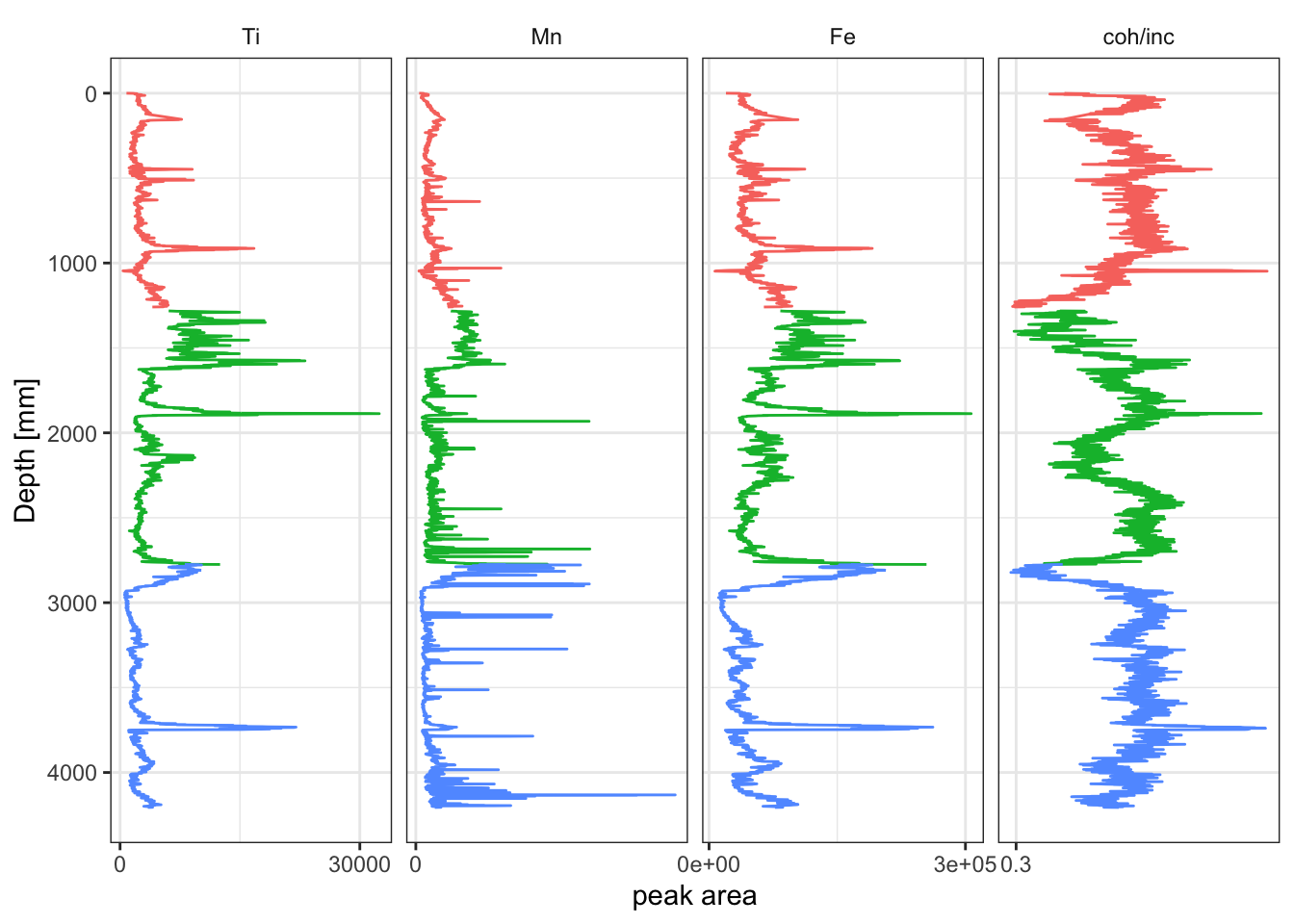## 4.1 Plotting XRF Data

For simple biplots, `ggplot2` provides the following solution. This includes different colour coding to differentiate the core sections.

``````ggplot(data = na.omit(CD166_19_xrf), mapping = aes(x = depth, y = `Mo coh`/`Mo inc`)) +
geom_line(aes(color = label)) +
coord_flip() +
scale_x_reverse() +
labs(x = "Depth [mm]", color = "Core") +
theme_classic() +
theme(legend.position = "none")``````Where a traditional stratigraphic plot is desired, the `tidypaleo` package provides useful functionality for producing these. However, the data does need to converted to long-form from the existing table. In this way `tidypaleo::facet_geochem_gridh` works in a way similar to `ggplot::facet_wrap` but has been creates a stratigraphic plot. The `elementsList` is used with `select(any_of())` to include only columns that are chemical elements. `Depth` and `label` are also added because they are used in the plot. Although this is useful for producing large-format summary diagrams of the data, these do not render well in smaller plot areas, so a small subset of variables has been selected manually; see the code comments below. Note that in the line `mutate()`, the vector passed to `levels =` controls the order in which the plots appear. In this example, they will appear in order of atomic weight, and the `coh/inc` ratio will always be last.

``````xrfStrat <- CD166_19_xrf %>%
mutate(`coh/inc` = `Mo coh`/`Mo inc`) %>%
select(any_of(elementsList), `coh/inc`, depth, label) %>% # this is useful but produces a very large plot that doesn't render well
select(Fe, Ti, `coh/inc`, Mn, depth, label) %>% # a smaller set of elements is defined here manually.
tidyr::pivot_longer(!c("depth", "label"), names_to = "elements", values_to = "peakarea") %>%
tidyr::drop_na() %>%
mutate(elements = factor(elements, levels = c(elementsList, "coh/inc"))) %>% # note that the levels controls the order

ggplot(aes(x = peakarea, y = depth)) +
tidypaleo::geom_lineh(aes(color = label)) +
scale_y_reverse() +
scale_x_continuous(n.breaks = 2) +
facet_geochem_gridh(vars(elements)) +
labs(x = "peak area", y = "Depth [mm]") +
tidypaleo::theme_paleo() +
theme(legend.position = "none")

print(xrfStrat)``````Notice that in the previous example the data must be converted to “long-form” from “short-form” for `tidypaleo`, using `tidyr::pivot_longer`. “Short-form” data has observations as rows, and variables as columns, whereas “long-form” data has a column for `names`, which in this case are the elements, and a column for `values`, in this case the peak intensities. This results in many rows, but few columns.

``````CD166_19_xrf %>%
select(any_of(elementsList), depth, label) %>%
tidyr::pivot_longer(!c("depth", "label"), names_to = "elements", values_to = "peakarea") %>%
glimpse()``````
``````## Rows: 151,632
## Columns: 4
## \$ depth    <dbl> 0, 0, 0, 0, 0, 0, 0, 0, 0, 0, 0, 0, 0, 0, 0, 0, 0, 0, 0, 0, 0…
## \$ label    <chr> "S1", "S1", "S1", "S1", "S1", "S1", "S1", "S1", "S1", "S1", "…
## \$ elements <chr> "Al", "Si", "P", "S", "Cl", "Ar", "K", "Ca", "Sc", "Ti", "V",…
## \$ peakarea <dbl> 41, 177, 0, 0, 726, 697, 1412, 59965, 19, 804, 26, 220, 231, …``````